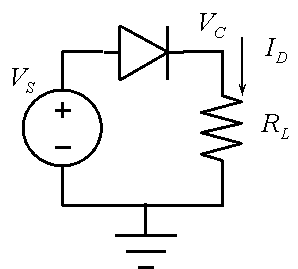# ECE446/ECE531 Microelectronics Assignment 2

1. For the diode circuit below, assume RL = 100 ohm, VS = 8 V, and the diode is 1N4148. Find the current ID using LTSpice.2. For the circuit, above, let the voltage source be a 16 V peak-to-peak sine wave with a frequency of 400 Hz. Plot the rectified voltage VL as a function of time for several cycles, using LTSpice.

3. A simple diode test circuit was simulated in class (pdf). Repeat the simulation using IS=1E-16, TT=25e-9, and VJ=0.75 in the diode model parameters. Find the forward and reverse bias current and the diode storage time. Download a starting version of mylib.lib.

4. Work through tutorial 1 (see link below). Your report should include a layout figure, Spice circuit figure, and a report of Spice analysis for the voltage divider.

Tutorial 1 – Layout and simulation of a resistive voltage divider – electric_tutorial_1_video.wmv (27:57)

5. Explain the terms crosstalk and ground bounce as applied to microelectronic circuit design. Reference the sources you use.

6. What is a Schottky diode? What is a Zener diode? How do they compare to a silicon diode? Reference the sources you use.

Maintained by John Loomis, last updated 22 January 2015